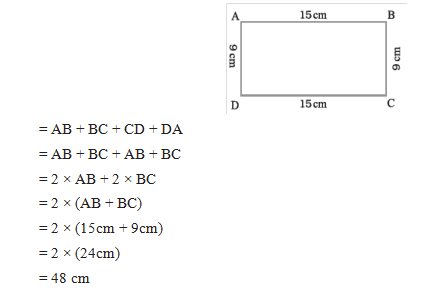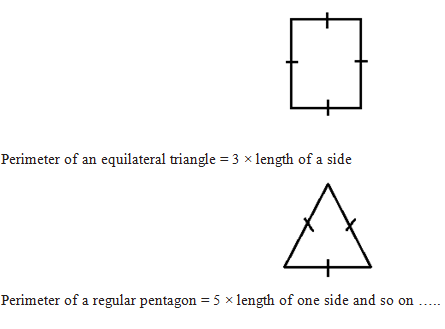# Perimeter

## Mensuration of Class 6

### Perimeter

Perimeter is the distance covered along the boundary forming a closed figure when you go round the figure once.

### Perimeter of a rectangle

Perimeter of the rectangle = Sum of the lengths of its four sides.

Perimeter of a rectangle = length + breadth + length + breadth

i.e. Perimeter of a rectangle = 2 × (length + breadth)Illustration 1:

An athlete takes 10 rounds of a rectangular park, 50 m long and 25 m wide. Find the total distance covered by him.

Sol.      Length of the rectangular park = 50 m

Breadth of the rectangular park = 25 m

Total distance covered by the athlete in one round will be the perimeter of the park.

Now, perimeter of the rectangular park

= 2 × (length + breadth) = 2 × (50 m + 25 m)

= 2 × 75 m = 150 m

So, the distance covered by the athlete in one round is 150 m.

Therefore, distance covered in 10 rounds = 10 × 150 m = 1500m

The total distance covered by the athlete is 1500 m.

### Perimeter of regular shapes

Perimeter of a square = 4 × length of a sideIllustration 2:

Find the perimeter of a regular pentagon with each side measuring 3 cm.

Sol.      This regular closed figure has 5 sides, each with a length of 3 cm.

Thus, we get

Perimeter of the regular pentagon = 5 × 3 cm = 15 cm

Illustration 3:

The perimeter of a regular hexagon is 18 cm. How long is its one side?

Sol.      Perimeter = 18 cm

A regular hexagon has 6 sides, so we can divide the perimeter by 6 to get the length of one side.

One side of the hexagon = 18 cm ÷ 6 = 3 cm

Therefore, length of each side of the regular hexagon is 3 cm.